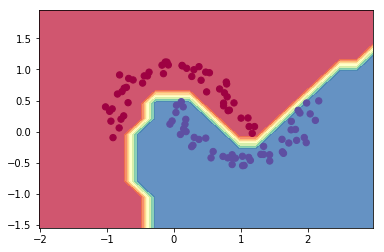A tiny scalar-valued autograd engine and a neural net library on top of it with PyTorch-like API
Pytorch71,0773,3416,72816 hours ago37May 08, 202312,759otherPython
Tensors and Dynamic neural networks in Python with strong GPU acceleration
Pytorch Tutorial27,137
a month ago85mitPython
PyTorch Tutorial for Deep Learning Researchers
Examples20,784
24 days ago185bsd-3-clausePython
A set of examples around pytorch in Vision, Text, Reinforcement Learning, etc.
Awesome Pytorch List14,103
4 months ago4
A comprehensive list of pytorch related content on github,such as different models,implementations,helper libraries,tutorials etc.
Nlp Tutorial12,403
6 months ago34mitJupyter Notebook
Natural Language Processing Tutorial for Deep Learning Researchers
Pytorch Book10,517
10 months ago133mitJupyter Notebook
PyTorch tutorials and fun projects including neural talk, neural style, poem writing, anime generation (《深度学习框架PyTorch：入门与实战》)
The Incredible Pytorch9,479
7 months ago1mit
The Incredible PyTorch: a curated list of tutorials, papers, projects, communities and more relating to PyTorch.
Pytorch Tutorial7,372
6 months ago28mitJupyter Notebook
Build your neural network easy and fast, 莫烦Python中文教学
Stanza6,78328717 hours ago18March 14, 202378otherPython
Stanford NLP Python library for tokenization, sentence segmentation, NER, and parsing of many human languages
Micrograd6,116221 days ago1April 18, 202028mitJupyter Notebook
A tiny scalar-valued autograd engine and a neural net library on top of it with PyTorch-like API
Select To Compare

Alternative Project ComparisonsA tiny Autograd engine (with a bite! :)). Implements backpropagation (reverse-mode autodiff) over a dynamically built DAG and a small neural networks library on top of it with a PyTorch-like API. Both are tiny, with about 100 and 50 lines of code respectively. The DAG only operates over scalar values, so e.g. we chop up each neuron into all of its individual tiny adds and multiplies. However, this is enough to build up entire deep neural nets doing binary classification, as the demo notebook shows. Potentially useful for educational purposes.

### Installation

``````pip install micrograd
``````

### Example usage

Below is a slightly contrived example showing a number of possible supported operations:

``````from micrograd.engine import Value

a = Value(-4.0)
b = Value(2.0)
c = a + b
d = a * b + b**3
c += c + 1
c += 1 + c + (-a)
d += d * 2 + (b + a).relu()
d += 3 * d + (b - a).relu()
e = c - d
f = e**2
g = f / 2.0
g += 10.0 / f
print(f'{g.data:.4f}') # prints 24.7041, the outcome of this forward pass
g.backward()
print(f'{a.grad:.4f}') # prints 138.8338, i.e. the numerical value of dg/da
print(f'{b.grad:.4f}') # prints 645.5773, i.e. the numerical value of dg/db
``````

### Training a neural net

The notebook `demo.ipynb` provides a full demo of training an 2-layer neural network (MLP) binary classifier. This is achieved by initializing a neural net from `micrograd.nn` module, implementing a simple svm "max-margin" binary classification loss and using SGD for optimization. As shown in the notebook, using a 2-layer neural net with two 16-node hidden layers we achieve the following decision boundary on the moon dataset:### Tracing / visualization

For added convenience, the notebook `trace_graph.ipynb` produces graphviz visualizations. E.g. this one below is of a simple 2D neuron, arrived at by calling `draw_dot` on the code below, and it shows both the data (left number in each node) and the gradient (right number in each node).

``````from micrograd import nn
n = nn.Neuron(2)
x = [Value(1.0), Value(-2.0)]
y = n(x)
dot = draw_dot(y)
``````### Running tests

To run the unit tests you will have to install PyTorch, which the tests use as a reference for verifying the correctness of the calculated gradients. Then simply:

``````python -m pytest
``````

MIT

Popular Neural Projects
Popular Pytorch Projects
Popular Machine Learning Categories
Related Searches

Get A Weekly Email With Trending Projects For These Categories
No Spam. Unsubscribe easily at any time.
Jupyter Notebook
Pytorch
Neural
Dag